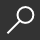## MATLAB for Dummies by Jim Sizemore and John Paul Mueller

Overview -
Plot graphs, solve equations, and write code in a flash

If you work in a STEM field, chances are you'll be using MATLAB on a daily basis. MATLAB is a popular and powerful computational tool and this book provides everything you need to start manipulating and plotting your data. MATLAB has rapidly become the premier data tool, and MATLAB For Dummies is a comprehensive guide to the fundamentals. MATLAB For Dummies guides you through this complex computational language from installation to visualization to automation.

• Learn MATLAB's language fundamentals including syntax, operators, and data types
• Understand how to use the most important window in MATLAB - the Command Window
• Get the basics of linear algebra to get up and running with vectors, matrices, and hyperspace
• Automate your work with programming scripts and functions
• Plot graphs in 2D and 3D to visualize your data
• Includes a handy guide for MATLAB's functions and plotting routines

MATLAB is an essential part of the analysis arsenal and MATLAB For Dummies provides clear, thorough guidance to get the most out of your data.

local_shippingFor DeliveryIn Stock.
FREE Shipping for Club Members helpNew & Used Marketplace 42 copies from \$4.80

## More About MATLAB for Dummies by Jim Sizemore; John Paul Mueller

### Overview

Plot graphs, solve equations, and write code in a flash

If you work in a STEM field, chances are you'll be using MATLAB on a daily basis. MATLAB is a popular and powerful computational tool and this book provides everything you need to start manipulating and plotting your data. MATLAB has rapidly become the premier data tool, and MATLAB For Dummies is a comprehensive guide to the fundamentals. MATLAB For Dummies guides you through this complex computational language from installation to visualization to automation.

• Learn MATLAB's language fundamentals including syntax, operators, and data types
• Understand how to use the most important window in MATLAB - the Command Window
• Get the basics of linear algebra to get up and running with vectors, matrices, and hyperspace
• Automate your work with programming scripts and functions
• Plot graphs in 2D and 3D to visualize your data
• Includes a handy guide for MATLAB's functions and plotting routines

MATLAB is an essential part of the analysis arsenal and MATLAB For Dummies provides clear, thorough guidance to get the most out of your data.

### Details

• ISBN-13: 9781118820100
• ISBN-10: 111882010X
• Publisher: For Dummies
• Publish Date: November 2014
• Page Count: 432
• Dimensions: 9.26 x 7.4 x 0.87 inches
• Shipping Weight: 1.25 poundsRelated Categories